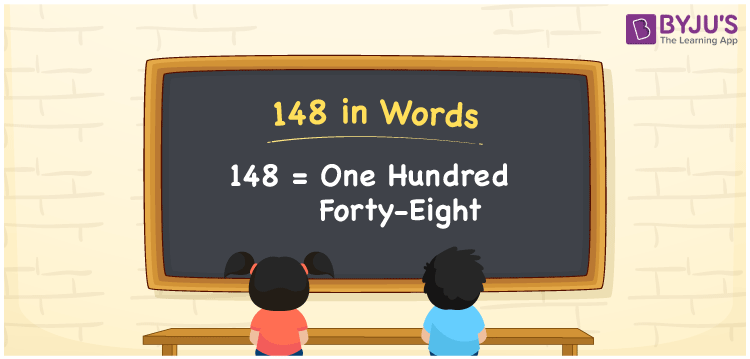# 148 in words

148 in words is written as One Hundred and Forty Eight. 148 represents the count or value. The article on Counting Numbers can give you an idea about count or counting. The number 148 is a 3 digit number that is used in expressions related to money, days, distance, length, weight and so on. Let us consider an example for 148. “There are One Hundred and Forty Eight people to be saved before the volcano erupts.”

 148 in words One Hundred and Forty Eight One Hundred and Forty Eight in Numbers 148

## 148 in English Words## How to Write 148 in Words?

We can convert 148 to words using a place value chart. The number 148 has 3 digits, so let’s make a chart that shows the place value up to 3 digits.

 Hundreds Tens Ones 1 4 8

Thus, we can write the expanded form as:

1 × Hundred + 4 × Ten + 8 × One

= 1 × 100 + 4 × 10 + 8 × 1

= 148

= One Hundred and Forty Eight.

148 is the natural number that is succeeded by 147 and preceded by 149.

148 in words – One Hundred and Forty Eight.

Is 148 an odd number? – No.

Is 148 an even number? – Yes.

Is 148 a perfect square number? – No.

Is 148 a perfect cube number? – No.

Is 148 a prime number? – No.

Is 148 a composite number? – Yes.

## Solved Example

1. Write the number 148 in expanded form

Solution: 1 × 100 + 4 × 10 + 8 × 1

We can write 148 = 100 + 40 + 8

= 1 × 100 + 4 × 10 + 8 × 1.

## Frequently Asked Questions on 148 in words

Q1

### How to write the number 148 in words?

148 in words is written as One Hundred and Forty Eight.
Q2

### Is 148 divisible by 3?

No. 148 is not divisible by 3.
Q3

### Is 148 a perfect square number?

No. 148 is not a perfect square number.